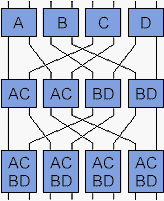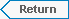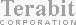Group TheoryGroup Theory is the mathematical study of permutations. The joke about Group Theory is that the only "practical" uses for Group Theory are in high energy physics and card tricks. One of the classic text book examples is a "grand tour" permutation. It shows how a set of elements can be permuted in a fixed manner and eventually return to its original order. One obvious permutation that will accomplish this is a right cyclic shift of the elements. An N element set will repeat itself after N applications of this permutation. What is not obvious is that there are permutations that will repeat the original set order after only log 2  of N applications. This example shows how such a permutation can repeat the original order of an 8 element set after only 3 applications.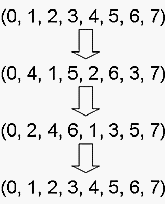There is also a card trick equivalent to this permutation called a "perfect shuffle." The order of the deck will repeat after log 2 N shuffles. 1) Select 8 cards; 2) Deal the first 4 into a first pile; 3) Deal the last 4 card into a second pile; 4) Deal the bottom card of the first pile onto a third pile; 5) Deal the bottom card of the second pile onto the third pile; 6) Repeat steps 4 and 5 until the first and second piles are depleted; 7) Take the third pile and repeat steps 2 through 6; and 8) Take the third pile and repeat steps 2 through 6.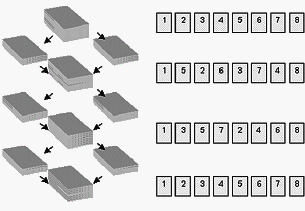The mathematical permutation and card trick can also be represented spatially as a set of mappings.The "grand tour" or "perfect shuffle" has a special property that can be best be seen spatially. These permutations are also topologically complete. In other words, all the inputs can reach all the outputs.